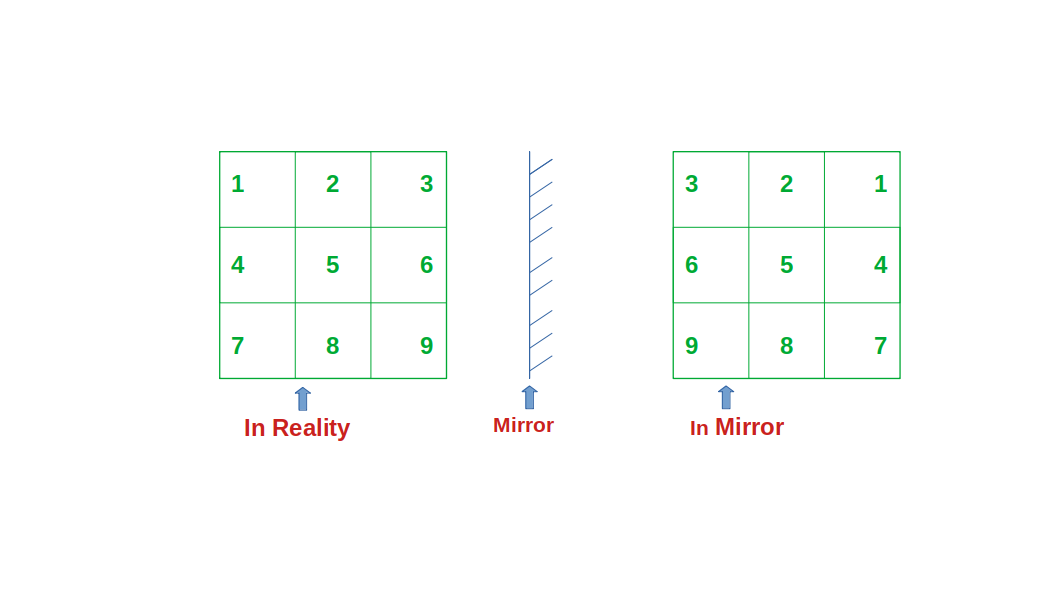Related Articles

# Check if the given two matrices are mirror images of one another

• Difficulty Level : Medium
• Last Updated : 23 Apr, 2021

Given two matrices mat1[][] and mat2[][] of size NxN. The task is to find if the given two matrices are mirror images of one another. Print “Yes” if the given two matrices are mirror images, otherwise print “No”.

Two matrices mat1 and mat2 of size N*N are said to be mirror images of one another if for any valid index (i, j) of the matrix:

`mat1[i][j] = mat2[i][N-j-1]`Examples:

Input:
mat1[][] = {{1, 2, 3}, {3, 4, 5}, {6, 7, 8}},
mat2[][] = {{3, 2, 1}, {5, 4, 3}, {8, 7, 6}}
Output: Yes

Input:
mat1 = {{1, 2, 3}, {5, 4, 1}, {6, 7, 2}};
mat2 = {{3, 2, 1}, {5, 4, 1}, {2, 7, 6}};
Output: No

Approach: The approach is based on the observation that a matrix is a mirror image of another only if the elements of each row of the first matrix are equal to the reversed row of elements of the other matrix. Follow the steps below :

• Traverse over the matrix mat1[][] row-wise from start to end and over mat2[][] row-wise from end to start.
• While traversing, if any element of mat1[][] is found to be not equal to the element at mat2[][], then print “No”
• After traversing both the matrices, if all the elements are found to be equal, print “Yes”.

Below is the implementation of the above approach:

## C++

 `// C++ implementation of``// the above approach``#include ``using` `namespace` `std;` `// Function to check whether the``// two matrices are mirror``// of each other``void` `mirrorMatrix(``int` `mat1[],``                  ``int` `mat2[], ``int` `N)``{``    ``// Initialising row and column of``    ``// second matrix``    ``int` `row = 0;``    ``int` `col = 0;` `    ``bool` `isMirrorImage = ``true``;` `    ``// Iterating over the matrices``    ``for` `(``int` `i = 0; i < N; i++) {` `        ``// Check row of first matrix with``        ``// reversed row of second matrix``        ``for` `(``int` `j = N - 1; j >= 0; j--) {` `            ``// If the element is not equal``            ``if` `(mat2[row][col] != mat1[i][j]) {``                ``isMirrorImage = ``false``;``            ``}` `            ``// Increment column``            ``col++;``        ``}` `        ``// Reset column to 0``        ``// for new row``        ``col = 0;` `        ``// Increment row``        ``row++;``    ``}` `    ``if` `(isMirrorImage)``        ``cout << ``"Yes"``;``    ``else``        ``cout << ``"No"``;``}` `// Driver code``int` `main()``{``    ``// Given 2 matrices``    ``int` `N = 4;``    ``int` `mat1[] = { { 1, 2, 3, 4 },``                      ``{ 0, 6, 7, 8 },``                      ``{ 9, 10, 11, 12 },``                      ``{ 13, 14, 15, 16 } };` `    ``int` `mat2[] = { { 4, 3, 2, 1 },``                      ``{ 8, 7, 6, 0 },``                      ``{ 12, 11, 10, 9 },``                      ``{ 16, 15, 14, 13 } };` `    ``// Function Call``    ``mirrorMatrix(mat1, mat2, N);``}`

## Java

 `// Java implementation of``// the above approach``import` `java.util.*;``class` `GFG{` `// Function to check whether the``// two matrices are mirror``// of each other``static` `void` `mirrorMatrix(``int` `mat1[][],``                         ``int` `mat2[][], ``int` `N)``{``  ``// Initialising row and column of``  ``// second matrix``  ``int` `row = ``0``;``  ``int` `col = ``0``;` `  ``boolean` `isMirrorImage = ``true``;` `  ``// Iterating over the matrices``  ``for` `(``int` `i = ``0``; i < N; i++)``  ``{``    ``// Check row of first matrix with``    ``// reversed row of second matrix``    ``for` `(``int` `j = N - ``1``; j >= ``0``; j--)``    ``{``      ``// If the element is not equal``      ``if` `(mat2[row][col] != mat1[i][j])``      ``{``        ``isMirrorImage = ``false``;``      ``}` `      ``// Increment column``      ``col++;``    ``}` `    ``// Reset column to 0``    ``// for new row``    ``col = ``0``;` `    ``// Increment row``    ``row++;``  ``}` `  ``if` `(isMirrorImage)``    ``System.out.print(``"Yes"``);``  ``else``    ``System.out.print(``"No"``);``}` `// Driver code``public` `static` `void` `main(String[] args)``{``  ``// Given 2 matrices``  ``int` `N = ``4``;``  ``int` `mat1[][] = {{``1``, ``2``, ``3``, ``4``},``                  ``{``0``, ``6``, ``7``, ``8``},``                  ``{``9``, ``10``, ``11``, ``12``},``                  ``{``13``, ``14``, ``15``, ``16``}};` `  ``int` `mat2[][] = {{``4``, ``3``, ``2``, ``1``},``                  ``{``8``, ``7``, ``6``, ``0``},``                  ``{``12``, ``11``, ``10``, ``9``},``                  ``{``16``, ``15``, ``14``, ``13``}};` `  ``// Function Call``  ``mirrorMatrix(mat1, mat2, N);``}``}` `// This code is contributed by 29AjayKumar`

## Python3

 `# Python3 implementation of``# the above approach` `# Function to check whether the``# two matrices are mirror``# of each other``def` `mirrorMatrix(mat1, mat2, N):` `    ``# Initialising row and column of``    ``# second matrix``    ``row ``=` `0``    ``col ``=` `0` `    ``isMirrorImage ``=` `True` `    ``# Iterating over the matrices``    ``for` `i ``in` `range``(N):` `        ``# Check row of first matrix with``        ``# reversed row of second matrix``        ``for` `j ``in` `range``(N ``-` `1``, ``-``1``, ``-``1``):` `            ``# If the element is not equal``            ``if` `(mat2[row][col] !``=` `mat1[i][j]):``                ``isMirrorImage ``=` `False` `            ``# Increment column``            ``col ``+``=` `1` `        ``# Reset column to 0``        ``# for new row``        ``col ``=` `0` `        ``# Increment row``        ``row ``+``=` `1` `    ``if` `(isMirrorImage):``        ``print``(``"Yes"``)``    ``else``:``        ``print``(``"No"``)` `# Driver code``if` `__name__ ``=``=` `'__main__'``:``    ` `    ``# Given 2 matrices``    ``N ``=` `4``    ``mat1 ``=` `[ [ ``1``, ``2``, ``3``, ``4` `],``             ``[ ``0``, ``6``, ``7``, ``8` `],``             ``[ ``9``, ``10``, ``11``, ``12` `],``             ``[ ``13``, ``14``, ``15``, ``16` `] ]` `    ``mat2 ``=` `[ [ ``4``, ``3``, ``2``, ``1` `],``             ``[ ``8``, ``7``, ``6``, ``0` `],``             ``[ ``12``, ``11``, ``10``, ``9` `],``             ``[ ``16``, ``15``, ``14``, ``13` `] ]` `    ``# Function call``    ``mirrorMatrix(mat1, mat2, N)` `# This code is contributed by mohit kumar 29`

## C#

 `// C# implementation of``// the above approach``using` `System;``class` `GFG{` `// Function to check whether the``// two matrices are mirror``// of each other``static` `void` `mirrorMatrix(``int``[,] mat1,``                         ``int` `[,]mat2, ``int` `N)``{``  ``// Initialising row and column of``  ``// second matrix``  ``int` `row = 0;``  ``int` `col = 0;` `  ``bool` `isMirrorImage = ``true``;` `  ``// Iterating over the matrices``  ``for` `(``int` `i = 0; i < N; i++)``  ``{``    ``// Check row of first matrix with``    ``// reversed row of second matrix``    ``for` `(``int` `j = N - 1; j >= 0; j--)``    ``{``      ``// If the element is not equal``      ``if` `(mat2[row, col] != mat1[i, j])``      ``{``        ``isMirrorImage = ``false``;``      ``}` `      ``// Increment column``      ``col++;``    ``}` `    ``// Reset column to 0``    ``// for new row``    ``col = 0;` `    ``// Increment row``    ``row++;``  ``}` `  ``if` `(isMirrorImage)``    ``Console.Write(``"Yes"``);``  ``else``    ``Console.Write(``"No"``);``}` `// Driver code``public` `static` `void` `Main(String[] args)``{``  ``// Given 2 matrices``  ``int` `N = 4;``  ``int` `[,]mat1 = {{1, 2, 3, 4},``                 ``{0, 6, 7, 8},``                 ``{9, 10, 11, 12},``                 ``{13, 14, 15, 16}};` `  ``int` `[,]mat2 = {{4, 3, 2, 1},``                 ``{8, 7, 6, 0},``                 ``{12, 11, 10, 9},``                 ``{16, 15, 14, 13}};` `  ``// Function Call``  ``mirrorMatrix(mat1, mat2, N);``}``}` `// This code is contributed by shikhasingrajput`

## Javascript

 ``
Output:
`Yes`

Time complexity: O(N2
Auxiliary Space: O(1)

Attention reader! Don’t stop learning now. Get hold of all the important DSA concepts with the DSA Self Paced Course at a student-friendly price and become industry ready.  To complete your preparation from learning a language to DS Algo and many more,  please refer Complete Interview Preparation Course.

In case you wish to attend live classes with experts, please refer DSA Live Classes for Working Professionals and Competitive Programming Live for Students.

My Personal Notes arrow_drop_up